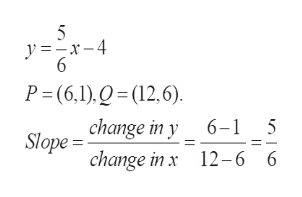I need help finding the slope of this equation y=5/6x−4

Question
I need help finding the slope of this equation

y=5/6x−4
Step 1

To determine the slope for the straight line with equation y = (5/6)x -4

Step 2

The slope of a straight line is just the rate of change of y with respect to x. One way to determine the slope is to locate two convenient...help_outlineImage Transcriptionclose5 y=x-4 6 P (6,1),Q(12,6). change in y 6-1 5 Slope change in x 12-6 6 fullscreen

Want to see the full answer?

See Solution

Want to see this answer and more?

Our solutions are written by experts, many with advanced degrees, and available 24/7

See Solution
Tagged in

Equations and In-equations